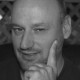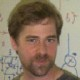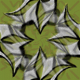## Is Life Improbable?

Mine? Yes. And maybe you’ve wondered just how improbable your life is. But that’s not really the question today…

Here at the Centre for Quantum Technologies, Dagomir Kaszlikowski asked me to give a talk on this paper:

• John Baez, Is life improbable?, Foundations of Physics 19 (1989), 91-95.

This was the second paper I wrote, right after my undergraduate thesis. Nobody ever seemed to care about it, so it’s strange—but nice—to finally be giving a talk on it.

My paper does not try to settle the question its title asks. Rather, it tries to refute the argument here:

• Eugene P. Wigner, The probability of the existence of a self-reproducing unit, Symmetries and Reflections, Indiana University Press, Bloomington, 1967, pp. 200-208.

According Wigner, his argument

purports to show that, according to standard quantum mechanical theory, the probability is zero for the existence of self-reproducing states, i.e., organisms.

Given how famous Eugene Wigner is (he won a Nobel prize, after all) and how earth-shattering his result would be if true, it’s surprising how little criticism his paper has received. David Bohm mentioned it approvingly in 1969. In 1974 Hubert Yockey cited it saying

for all physics has to offer, life should never have appeared and if it ever did it would soon die out.

As you’d expect, there are some websites mentioning Wigner’s argument as evidence that some supernatural phenomenon is required to keep life going. Wigner himself believed it was impossible to formulate quantum theory in a fully consistent way without referring to consciousness. Since I don’t believe either of these claims, I think it’s good to understand the flaw in Wigner’s argument.

So, let me start by explaining his argument. Very roughly, it purports to show that if there are many more ways a chunk of matter can be ‘dead’ than ‘living’, the chance is zero that we can choose some definition of ‘living’ and a suitable ‘nutrient’ state such that every ‘living’ chunk of matter can interact with this ‘nutrient’ state to produce two ‘living’ chunks.

In making this precise, Wigner considers more than just two chunks of matter: he also allows there to be an ‘environment’. So, he considers a quantum system made of three parts, and described by a Hilbert space$H = H_1 \otimes H_1 \otimes H_2$

Here the first$H_1$ corresponds to a chunk of matter. The second$H_1$ corresponds to another chunk of matter. The space$H_3$ corresponds to the ‘environment’. Suppose we wait for a certain amount of time and see what the system does; this will be described by some unitary operator$S: H \to H$

Wigner asks: if we pick this operator$S$ in a random way, what’s the probability that there’s some$n$-dimensional subspace of ‘living organism’ states in$H_1$, and some ‘nutrient plus environment’ state in$H_1 \otimes H_2$, such that the time evolution sends any living organism together with the nutrient plus environment to two living organisms and some state of the environment?

A bit more precisely: suppose we pick$S$ in a random way. Then what’s the probability that there exists an$n$-dimensional subspace$V \subseteq H_1$

and a state$w \in H_1 \otimes H_2$

such that$S$ maps every vector in$V \otimes \langle w \rangle$ to a vector in$V \otimes V \otimes H_2$? Here$\langle w \rangle$ means the 1-dimensional subspace spanned by the vector$w$.$\mathrm{dim}(H_1) \gg n$

then this probability is zero.

You may need to reread the last few paragraphs a couple times to understand Wigner’s question, and his answer. In case you’re still confused, I should say that$V \subseteq H_1$ is what I’m calling the space of ‘living organism’ states of our chunk of matter, while$w \in H_1 \otimes H_2$ is the ‘nutrient plus environment’ state.

Now, Wigner did not give a rigorous proof of his claim, nor did he say exactly what he meant by ‘probability’: he didn’t specify a probability measure on the space of unitary operators on$H$. But if we use the obvious choice (called ‘normalized Haar measure’) his argument can most likely be turned into a proof.

So, I don’t want to argue with his math. I want to argue with his interpretation of the math. He concludes that

the chances are nil for the existence of a set of ‘living’ states for which one can find a nutrient of such nature that interaction always leads to multiplication.

The problem is that he fixed the decomposition of the Hilbert space$H$ as a tensor product$H = H_1 \otimes H_1 \otimes H_2$

before choosing the time evolution operator$S$. There is no good reason to do that. It only makes sense split up a physical into parts this way after we have some idea of what the dynamics is. An abstract Hilbert space doesn’t come with a favored decomposition as a tensor product into three parts!

If we let ourselves pick this decomposition after picking the operator$S$, the story changes completely. My paper shows:

Theorem 1. Let$H$,$H_1$ and$H_2$ be finite-dimensional Hilbert spaces with$H \cong H_1 \otimes H_1 \otimes H_2$. Suppose$S : H \to H$ is any unitary operator, suppose$V$ is any subspace of$H_1$, and suppose$w$ is any unit vector in$H_1 \otimes H_2$ Then there is a unitary isomorphism$U: H \to H_1 \otimes H_1 \otimes H_2$

such that if we identify$H$ with$H_1 \otimes H_1 \otimes H_2$ using$U$, the operator$S$ maps$V \otimes \langle w \rangle$ into$V \otimes V \otimes H_2$.

In other words, if we allow ourselves to pick the decomposition after picking$S$, we can always find a ‘living organism’ subspace of any dimension we like, together with a ‘nutrient plus environment’ state that allows our living organism to reproduce.

However, if you look at the proof in my paper, you’ll see it’s based on a kind of cheap trick (as I forthrightly admit). Namely, I pick the ‘nutrient plus environment’ state to lie in$V \otimes H_2$, so the nutrient actually consists of another organism!

This goes to show that you have to be very careful about theorems like this. To prove that life is improbable, you need to find some necessary conditions for what counts as life, and show that these are improbable (in some sense, and of course it matters a lot what that sense is). Refuting such an argument does not prove that life is probable: for that you need some sufficient conditions for what counts as life. And either way, if you prove a theorem using a ‘cheap trick’, it probably hasn’t gotten to grips with the real issues.

I also show that as the dimension of$H$ approaches infinity, the probability approaches 1 that we can get reproduction with a 1-dimensional ‘living organism’ subspace and a ‘nutrient plus environment’ state that lies in orthogonal complement of$V \otimes H_2$. In other words, the ‘nutrient’ is not just another organism sitting there all ready to go!

More precisely:

Theorem 2. Let$H$,$H_1$ and$H_2$ be finite-dimensional Hilbert spaces with$\mathrm{dim}(H) = \mathrm{dim}(H_1)^2 \cdot \mathrm{dim}(H_2)$. Let$\mathbf{S'}$ be the set of unitary operators$S: H \to H$ with the following property: there’s a unit vector$v \in H_1$, a unit vector$w \in V^\perp \otimes H_2$, and a unitary isomorphism$U: H \to H_1 \otimes H_1 \otimes H_2$

such that if we identify$H$ with$H_1 \otimes H_1 \otimes H_2$ using$U$, the operator$S$ maps$v \otimes w$ into$\langle v\rangle \otimes \langle v \rangle \otimes H_2$. Then the normalized Haar measure of$\mathbf{S'}$ approaches 1 as$\mathrm{dim}(H) \to \infty$.

Here$V^\perp$ is the orthogonal complement of$V \subseteq H_1$; that is, the space of all vectors perpendicular to$V$.

I won’t include the proofs of these theorems, since you can see them in my paper.

Just to be clear: I certainly don’t think these theorems prove that life is probable! You can’t have theorems without definitions, and I think that coming up with a good general definition of ‘life’, or even supposedly simpler concepts like ‘entity’ and ‘reproduction’, is extremely tough. The formalism discussed here is oversimplified for dozens of reasons, a few of which are listed at the end of my paper. So far we’re only in the first fumbling stages of addressing some very hard questions.

All my theorems do is point out that Wigner’s argument has a major flaw: he’s choosing a way to divide the world into chunks of matter and the environment before choosing his laws of physics. This doesn’t make much sense, and reversing the order dramatically changes the conclusions.

By the way: I just started looking for post-1989 discussions of Wigner’s paper. So far I haven’t found any interesting ones. Here’s a more recent paper that’s somewhat related, which doesn’t mention Wigner’s work:

• Indranil Chakrabarty and Prashant, Non existence of quantum mechanical self replicating machine, 2005.

The considerations here seem more closely related to the Wooters–Zurek no-cloning theorem.

### 8 Responses to Is Life Improbable?

1.Uwe Stroinski says:

The hunt for the ‘self-reproducing’ state seems to be something like the hunt for the perpetual motion machine. Its so far non-existence should tell us something about nature. Also, the relation of such ‘self-reproducing’ states to biological life is not obvious. It seems more like an ambiguous usage of ‘self-reproducing’ than anything else.

•John Baez says:

My paper shows that it’s pathetically easy to find self-reproducing states if you phrase the problem a certain way. It’s very hard if you phrase the problem another way. The relevance to biology is indeed non-obvious: as someone pointed out in my talk today, organisms keep changing slightly, so we shouldn’t expect to find a fixed subspace of ‘living’ states$V \subseteq H_1$ that has$S: V \otimes \langle w \rangle \to V \otimes V \otimes H_2$

(where I’m using notation as in my blog post above). However, I think we need to study stupid over-idealized models to realize that they’re stupid and over-idealized and find ways to make them a bit less stupid and over-idealized.

2.Kaleberg says:

Wasn’t Wigner the guy with the unreasonable effectiveness of mathematics paper? That always struck me as odd because any real world that can do arithmetic can contain models or analogs of its own processes. Once these exist, situations in which they are effective will elucidate them. I find it much harder to imagine a world in which one cannot construct some kind of effective mathematics. How would one describe it? (Of course, if there are an infinite number of random worlds, there may be an infinite number of them without effective mathematics since I suppose there are more worlds than descriptions, but I’d still like to see a Wigner universe.)

I’m not really surprised he ran into a similar problem dealing with the probability of life. If you use a broad enough definition, any repeating process can be considered a life form, and each repetition a new generation. The probability of repeating, or at least approximately repeating processes, always strikes me as high, though we’d have to put up with a lot of rather boring life forms.

•John Baez says:

Kaleberg wrote:

Wasn’t Wigner the guy with the unreasonable effectiveness of mathematics paper?

Yes: Wigner won the Nobel prize for his work applying group theory to nuclear physics and particle physics, but later he started wondering why such abstract math was so good at describing our universe. You can see his famous essay here:

• Eugene Wigner The unreasonable effectiveness in the mathematical sciences, 1960.

It begins like this:

There is a story about two friends, who were classmates in high school, talking about their jobs. One of them became a statistician and was working on population trends. He showed a reprint to his former classmate. The reprint started, as usual, with the Gaussian distribution and the statistician explained to his former classmate the meaning of the symbols for the actual population, for the average population, and so on. His classmate was a bit incredulous and was not quite sure whether the statistician was pulling his leg. “How can you know that?” was his query. “And what is this symbol here?” “Oh,” said the statistician, “this is pi.” “What is that?” “The ratio of the circumference of the circle to its diameter.” “Well, now you are pushing your joke too far,” said the classmate, “surely the population has nothing to do with the circumference of the circle.”

Of course they were talking about the normal distribution$\displaystyle{ \frac{1}{\sqrt{2 \pi \sigma^2}} e^{-x^2 / 2 \sigma^2 }}$

(Of course, if there are an infinite number of random worlds, there may be an infinite number of them without effective mathematics since I suppose there are more worlds than descriptions, but I’d still like to see a Wigner universe.)

Greg Egan has nicely argued that if we weight simple worlds more heavily than complicated ones, than the most heavily weighted worlds with life in them are going to have nice mathematical descriptions. This idea of weighting worlds, i.e. hypotheses, according to their simplicity is the basis of Solomonoff induction, which Eliezer Yudkowsky mentioned in “week313” of This Week’s Finds.

However, nonmathematicians may find this bias towards simplicity gives math an unfair advantage.•Todd Trimble says:

The first of those two Wigner stories sounds exactly like a story told about Augustus De Morgan by W.W.R. Ball; see the third quote here.

3.Alexander Vlasov says:

Hello,

It seems to me, even if QM formally does not prohibit self-replication: http://arxiv.org/abs/quant-ph/0403037 it may not be done precisely…

4.Gilbert R. Sandgren says:

I am old, cannot do math anymore, and came accross this discussion largely by accident, but isn’t Wigner’s gesture here more like ‘Under existing physical laws it seems impossible that life would arise. Life arose. Ergo, some new laws are needed.’ Wigner’s formulation initially looks fine to me when we limit ourselves to the question of how/why life arose…

•John Baez says:

The problem is that Wigner’s argument shows only that for any particular very precisely defined form of life, it’s unlikely that randomly chosen laws of physics will let this particular form of life reproduce. The more interesting question is whether randomly chosen laws of physics will let some form of life reproduce.

This site uses Akismet to reduce spam. Learn how your comment data is processed.